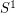Importance: Medium ✭✭
 Author(s): Georgakopoulos, Agelos
 Subject: Graph Theory » Infinite Graphs
 Keywords: hamiltonian infinite graph line graphs
 Posted by: Robert Samal on: July 24th, 2007
Conjecture
\item Ifis a 4-edge-connected locally finite graph, then its line graph is hamiltonian. \item If the line graphof a locally finite graphis 4-connected, thenis hamiltonian.

(Reproduced from [M].)

A locally finite graph is hamiltonian, if its Freudenthal compactification (also called the end compactification, see [D]) contains a hamilton circle, i.e. a homeomorphic copy ofcontaining all vertices.

The first part is known for finite graphs. The proof uses the existence of two edge-disjoint spanning trees in 4-edge-connected graphs. In the infinite case, it would be enough to prove that a 4-edge-connected locally finite graphhas two edge-disjoint topological spanning trees (see [D]), one of which is connected as a subgraph of. The problem is open even for the 1-ended case (where hamilton circles correspond to 2-way-infinite paths).

The second part is widely open even in the finite case, where it was proposed by Thomassen [T].

## Bibliography

[D] Reinhard Diestel, Graph Theory, Third Edition, Springer, 2005.

*[G] A. Georgakopoulos, Oberwolfach reports, 2007.

[M] Bojan Mohar, Problem of the Month

[T] Carsten Thomassen, Reflections on graph theory, J. Graph Theory 10 (1986) 309-324, MathSciNet

* indicates original appearance(s) of problem.